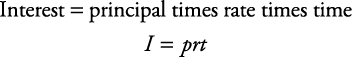## Simple Interest

##### Example 1

How much simple interest will an account earn in five years if \$500 is invested at 8% interest per year?

First, circle what you must find— interest. Now use the equationSimply plug into the equation.Note that both rate and time are in yearly terms (annual rate; years).